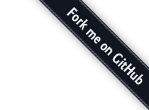``````(1 + 2) + 3      // a
``````

``````1 + (2 + 3)      // b
``````

`1 + 2 + 3` 简单的理解就是 “把1、2、3加在一起”， 确实，在我们接触到的数学里面，就是把三个数加起来。 但是在编程语言中，却不仅仅这样。

+4 是一个整数，但是 0+4 是一个加法表达式，这个表达式的求值结果正好是 +4

``````short b = 1;
short b = b + 4;   // 警告
short b += 4;      // 无警告
``````

1 + 2 是一个表达式，它的返回值是 3。 这个表达式的返回值再参加到另一个表达式中 3 + 3，最后得出结果6。

``````// 计算 1 + 2 + 3
var a = 1 + 2;
var b = b + 3;
``````

``````(+ (+ 1 2) 3)
``````

``````5 > 4 > 3
``````